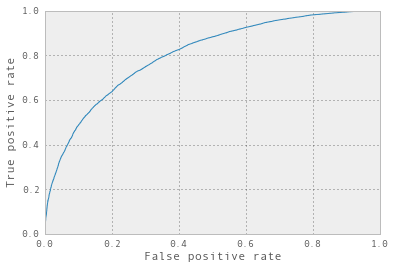# scikit learn in 3 lines of code except actually it's more like 20¶

First, we'll generate a dataset. It has two features called panda and elephant, and we generate a binary output target variable target.

In :
import pandas as pd
import numpy as np

In :
dataset = pd.DataFrame({
'panda': np.random.normal(0, 1, 100000),
'elephant': np.random.normal(0, 1, 100000)
})
x = - 1/3 * (dataset['panda'] +  dataset['elephant'])
transformed = 1 / (1 + np.exp(-1 * x))
dataset['target'] = np.random.uniform(0,1, 100000) < transformed
dataset.target.value_counts()

Out:
True     50032
False    49968
dtype: int64

Now that we have fake data, we can build a classifier!

In :
from sklearn.linear_model import LogisticRegression
from sklearn.cross_validation import train_test_split
from sklearn.metrics import confusion_matrix
cls = LogisticRegression()
features = dataset[['elephant', 'panda']]
target = dataset['target']
features_train, features_test, target_train, target_test = train_test_split(features, target)
cls.fit(features_train, target_train)
predictions = cls.predict(features_test)
print confusion_matrix(predictions, target_test)

[[8992 3512]
[3338 9158]]


So we have a classifier which is classifying 8953 + 9030 things correctly, and 3508 + 3500 things wrong. That's very good! That was legitimately something like 3 lines of code -- most of the work was actually setting up the fake dataset.

In :
pd.set_option('display.mpl_style', 'default')

In :
from sklearn.metrics import roc_curve
import matplotlib.pyplot as plt
scores = cls.predict_proba(features_test).transpose()
fpr, tpr, thresholds = roc_curve(target_test, scores)
plt.xlabel('False positive rate')
plt.ylabel('True positive rate')
plt.plot(fpr, tpr)

Out:
[<matplotlib.lines.Line2D at 0x7f3a004baa50>]